# Math Formula Worksheets

#### What are Math Formulas?

Math formulas are different than math expression. A formula will always an equality sign. Moreover, it will also have a conditional operator between them. So, in other words, it will have two or more variables which will be equal to different values. For instance, if we take the formula of volume; it is V = L × h × w. In this case, the value of L, h, and w could vary. Formulas are used to state rules or facts that contains values. Many times, we will either know or calculate one side of the formula. If we can do this, we can easily determine the other side of the formula because the overall outcome must be equal to that value.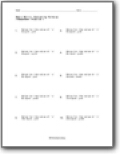###### Evaluating Formulas

We solve for the value of variables. For example: Solve for the value of 'x' in 4x + y = 0 ; y = 5.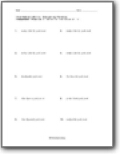###### Evaluating Thicker Formulas

These formulas contain multiple variables that you must work in. Example: x = 9y - (3z/3); y = 3, z = 4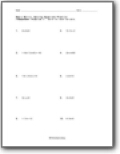###### Solving Equations Practice

Solve for each variable.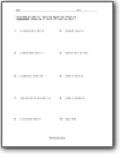###### Solving Decimal Based Equations

These equations involve both decimals and whole numbers.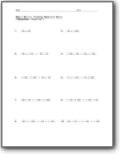###### Finding Absolute Value

These are expressions that require you to evaluate them with a terminal absolute value.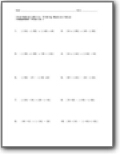###### Complex Absolute Value

These are a bit difficult for some students.